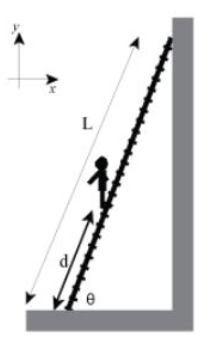# Problem: A uniform stationary ladder of length L = 3.7 m and mass M = 19 kg leans against a smooth vertical wall, while its bottom legs rest on a rough horizontal floor. The coefficient of static friction between floor and ladder is μ = 0.44. The ladder makes an angle θ = 56° with respect to the floor. A painter of mass 8M stands on the ladder a distance d from its base.Part (a) Find the magnitude of the normal force N, in newtons, exerted by the floor on the ladder. A numeric value is expected and not an expression.Part (b) Find an expression for the magnitude of the normal force Nw exerted by the wall on the ladder. Part (c) What is the largest distance up the ladder dmax, in meters, that the painter can stand without the ladder slipping? A numeric value is expected and not an expression.

###### FREE Expert Solution

Newton's second law:

$\overline{){\mathbf{\Sigma }}{\mathbf{F}}{\mathbf{=}}{\mathbf{m}}{\mathbf{a}}}$

Part (a)

The normal force exerted by the floor on the ladder is determined from the sum of all vertical forces. The normal force is directed upward while the downward forces are the weight of the ladder and weight of painter.

ΣF = N - Mg - 8Mg = 0

N = (M + 8M)g = 9Mg = (9)(19)(9.81)

85% (112 ratings)###### Problem Details

A uniform stationary ladder of length L = 3.7 m and mass M = 19 kg leans against a smooth vertical wall, while its bottom legs rest on a rough horizontal floor. The coefficient of static friction between floor and ladder is μ = 0.44. The ladder makes an angle θ = 56° with respect to the floor. A painter of mass 8M stands on the ladder a distance d from its base.Part (a) Find the magnitude of the normal force N, in newtons, exerted by the floor on the ladder. A numeric value is expected and not an expression.

Part (b) Find an expression for the magnitude of the normal force Nw exerted by the wall on the ladder.

Part (c) What is the largest distance up the ladder dmax, in meters, that the painter can stand without the ladder slipping? A numeric value is expected and not an expression.